Smartick is an advanced online program that teaches kids math and coding in only 15 min. a day
Try it for free!

Feb15

# How to Solve a Problem Involving Dividing 2 Digit Numbers

Do you remember your multiplication tables? Do you remember how to perform 1 digit divisions? Knowing how to do these things is important for being able to solve problems involving dividing 2 digit numbers.

Are you prepared to continue?

Before beginning, I am going to remind you of the different parts of a division problem since they will appear throughout the post.

• Dividend: The number that you have to divide.
• Divisor: The number that the dividend is being divided by.
• Quotient: The result of the division.
• Remainder: What is left over after the division.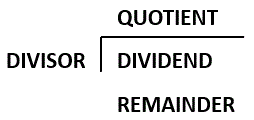Now we have to follow these steps to solve problems involving dividing 2 digit numbers:

1. Take the first digits of the dividend, the same number of digits that you have in the divisor. If the number that you have taken from the dividend is smaller than the divisor you have to take the next digit from the dividend.

In this example, we want to divide 9687 by 23. The divisor (23) has 2 digits so we have to take the first 2 digits from the dividend (96).

Since 96 is bigger than 23, we can divide.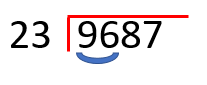1. Divide the first number of the dividend (or the first two numbers if in the previous step you had to take one more digit) by the first digit of the divisor. Write the result of this division in the part for the quotient.

The first digit of the dividend is 9 and the first digit of the divisor is 2, so we have to divide 9 by 2

9 / 2 = 4

We write the 4 in the quotient.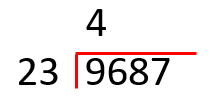1. Multiply the digit of the quotient by the divisor, write the result below the dividend and subtract the result from the dividend. If you cannot perform this subtraction because the dividend is smaller than the result, then you have to choose a smaller number in the quotient until you can subtract.

We multiply the quotient (4) by the divisor (23):

4 x 23 = 92

We write the result of the multiplication below the dividend (96) and subtract the two numbers:
96 – 92 = 4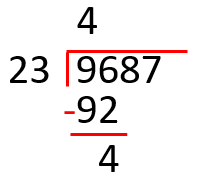1. Once you have done the subtraction, bring down the next digit of the dividend and continue to repeat the steps starting from step 2, until there are no more numbers in the dividend.

Now we bring down the next digit from the dividend (8).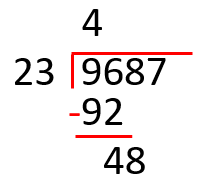Now we have to divide 48 by 23 repeating the same steps as before.

Would you know how to continue on your own?

We divide 48 by the divisor:
48 / 23 = 2

We write the 2 in the quotient and multiply it by the divisor:
2 x 23 = 46

We write the 46 below the dividend and subtract:
48 – 46 = 2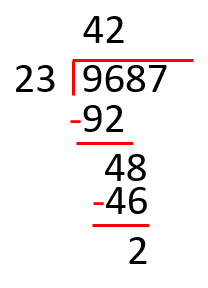We bring down the following digit: the 7

Now we have to divide 27 by 23:
27 / 23 = 1

We write the 1 in the quotient and multiply it by the divisor:
1 x 23 = 23

Now we subtract 27 – 23 = 4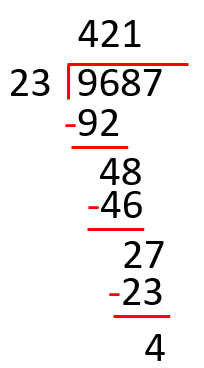Since there are no more digits left in the divisor we have already finished the division of the 2 numbers.

The result is 421 and the remainder is 4.

Have you learned to solve problems involving dividing 2 digit numbers? You should share this with your friends so they can learn too!

Learn More:

Latest posts by Smartick (see all)